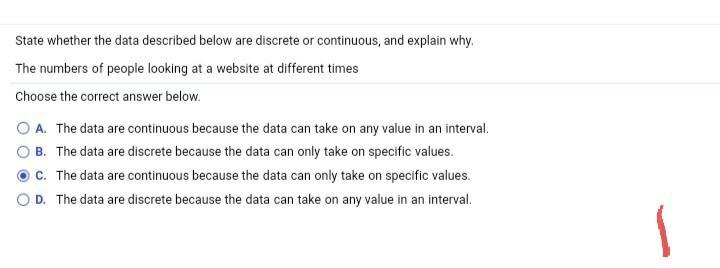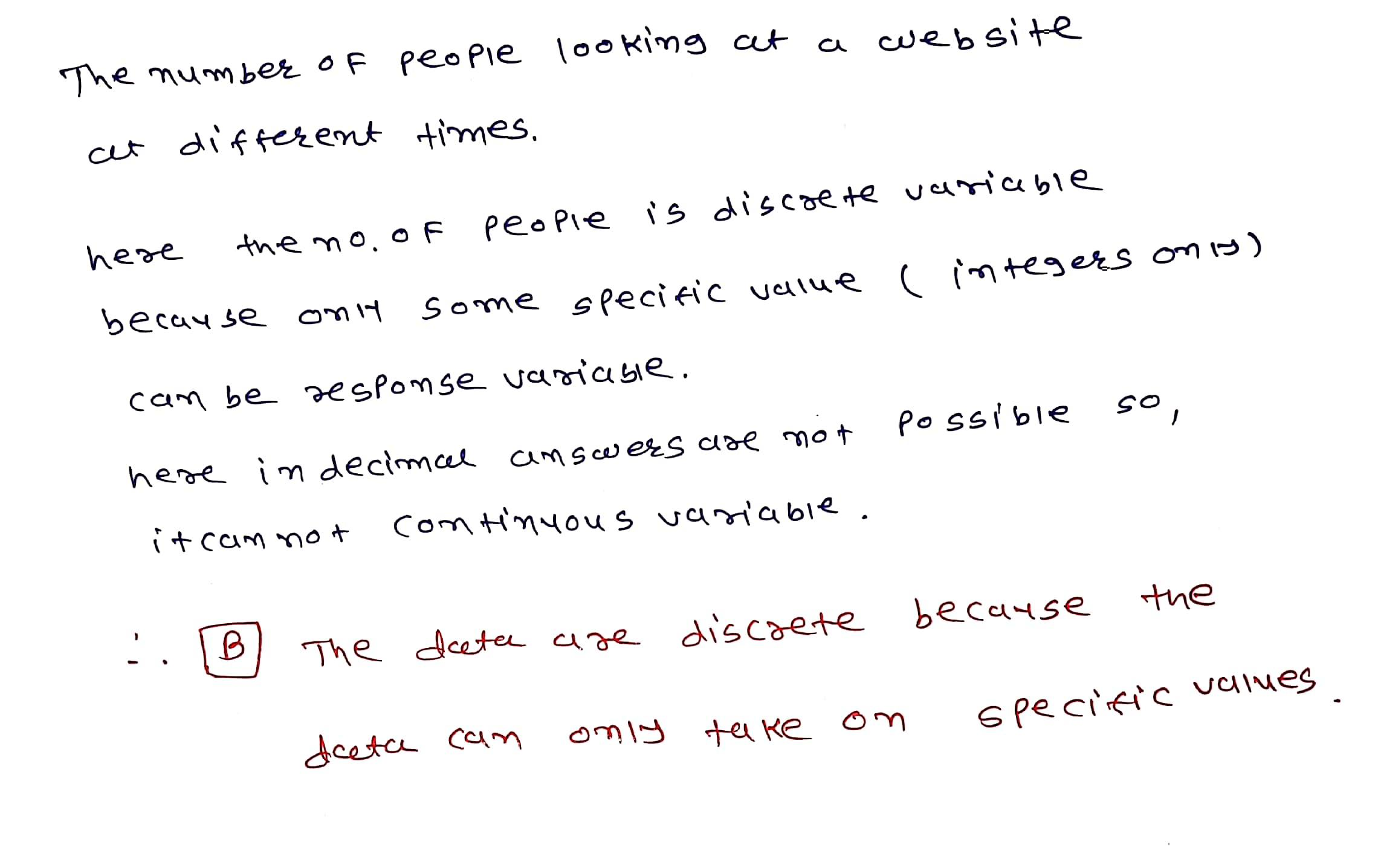# State whether the data described below are discrete or continuous. and explain why.please answer all parts State whether the data described below are discrete or continuous, and explain why, The numbers of people looking at a website at different times Choose the correct answer below. A. The data are continuous because the data can take on any value in an interval B. The data are discrete because the data can only take on specific values. C. The data are continuous because the data can only take on specific values. OD. The data are discrete because the data can take on any value in an interval

The number of people looking at a website cet different times. here the no. of people is discrete variable because only some specific value (integers on is) can be response variasie. possible so, here in decimcel answers are not itcam not continuous variable. the B The detae are discrete because specific values only take on deeta can